# Free Modules, Bases and Direct Sums/Products

Gold Member
I am reading Paul E. Bland's book "Rings and Their Modules ...

Currently I am focused on Section 2.2 Free Modules ... ...

I need some help in order to fully understand the proof of the equivalence of (1) and (3) in Proposition 2.2.3 ...

Proposition 2.2.3 and its proof reads as follows: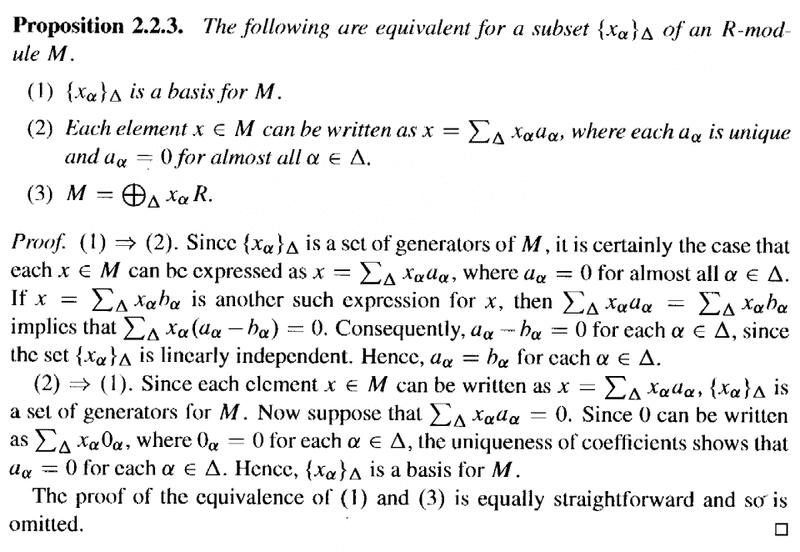Bland omits the proof of the equivalence of (1) and (3) ...

Can someone please help me to get started on a rigorous proof of the equivalence of (1) and (3) ... especially covering the case where $\Delta$ is an unaccountably infinite set ...

Peter

#### Attachments

andrewkirk
Homework Helper
Gold Member
I'm not familiar with Bland's book, so the notation seems somewhat unusual to me.

What does he mean by 'almost all ##\alpha\in\Delta##'? Does he mean 'all but a finite number', or is there some measure in operation here (which would be surprising in a book in modules)?

What is ##\Delta##? Is it an index set for the putative basis? It seems likely to be so from the way he uses it, but it's best to be sure.

What does he mean by ##\bigoplus_\Delta##? It seems he probably means a direct sum but if so, we need his exact definition of direct sum to attempt proving ##1\Leftrightarrow 3## since there are many slightly different (but generally provably equivalent) definitions of direct sum available.

Gold Member
oh OK ... sorry ... should have provided the relevant text ...

Text coming up ... just scanning it now ...

Peter

Gold Member
I'm not familiar with Bland's book, so the notation seems somewhat unusual to me.

What does he mean by 'almost all ##\alpha\in\Delta##'? Does he mean 'all but a finite number', or is there some measure in operation here (which would be surprising in a book in modules)?

What is ##\Delta##? Is it an index set for the putative basis? It seems likely to be so from the way he uses it, but it's best to be sure.

What does he mean by ##\bigoplus_\Delta##? It seems he probably means a direct sum but if so, we need his exact definition of direct sum to attempt proving ##1\Leftrightarrow 3## since there are many slightly different (but generally provably equivalent) definitions of direct sum available.

Important text from Bland regarding direct sum notation is as follows: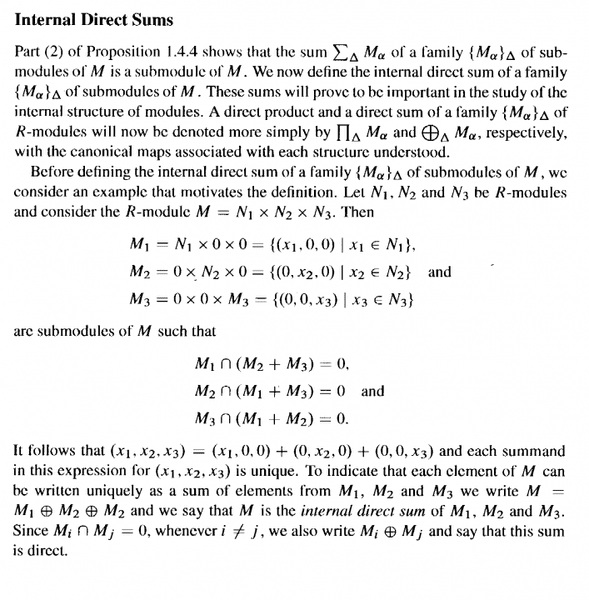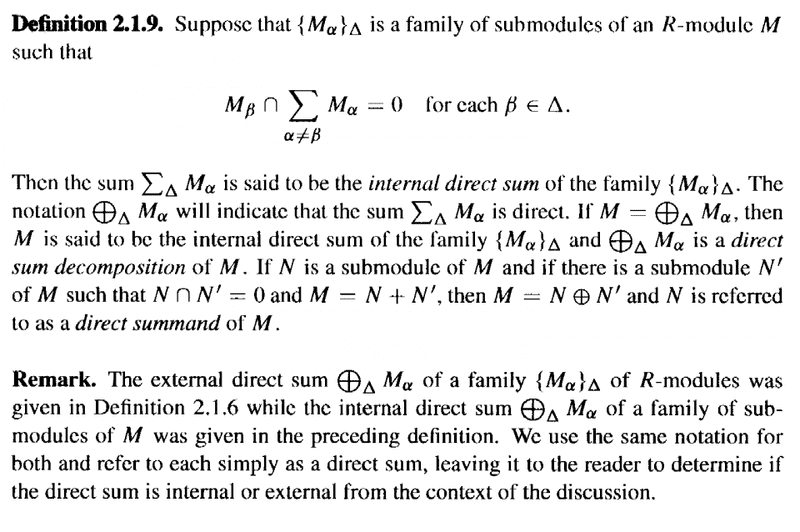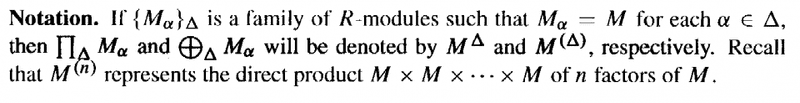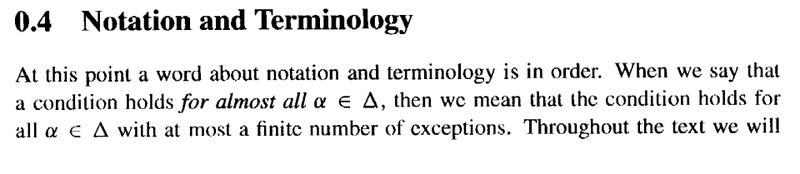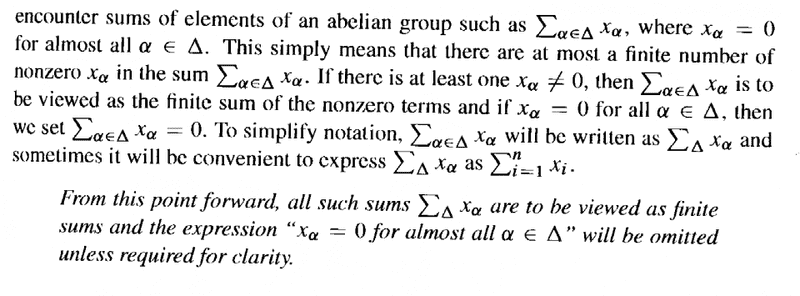#### Attachments

andrewkirk
Homework Helper
Gold Member
Thanks MA. That's as I expected, but it is best to be sure.

That being the case, here's one direction for you:

I prefer not to use an index set like $\Delta$ because it unnecessarily complicates notation. So let's instead set a name $B$ for the putative basis containing all the $x_\alpha$. I'll use $b$ for elements of the set, rather than $x_\alpha$. You can think of $B$ as corresponding to $\Delta$ except that it contains actual module elements rather than elements of some arbitrary other set that is not used for anything else.

We prove $3\Rightarrow 1$. If $m\in M$ then by (3) we know that $m$ can be written as a finite sum $m=\sum_{k=1}^r m_k$, where each $m_k$ is in a different module $b_kR$. So there exist $s_1,...,s_n\in R$ such that $\forall k\ m_k=b_ks_k$. Hence $m=\sum_{k=1}^n m_k=\sum_{k=1}^n b_ks_k$ which, being a finite linear combination of elements from $B$, means that it is in the module generated by $B$, and hence $B$ generates $M$.

Now linear independence. Say there exists $n\in\mathbb N$ and finite collections $\{b_1,...,b_n\}$ and $\{s_1,...,s_n\}$ of elements of $B$ and $R$ respectively such that $\sum_{k=1}b_ks_k=0$. We want to prove that all the summands are zero. For any $k'\in \{1,...,n\}$ we can rewrite the sum equation as $-b_k's_k'=\sum_{\substack{k=1\\k\neq k'}}b_ks_k$. The LHS of that is in the submodule $b_kR$ and the RHS is in the submodule $\sum_{\substack{k=1\\k\neq k'}}b_kR$. Since the direct sum property requires that the intersection of those two submodules is $\{0\}$, we conclude that $b_k's_k'=0$. Since that was an arbitrary summand, we conclude that all summands are zero. Hence there is no nontrivial linear combination of the $b$s that is zero, hence they are linearly independent.

So $B$ is a basis for $M$ and we have proven $3\Rightarrow 1$.

Think over this one and see if it gives you ideas for proving the other direction. If you feel you need help, let me know.

Last edited:
•Math Amateur
Gold Member
Thanks MA. That's as I expected, but it is best to be sure.

That being the case, here's one direction for you:

I prefer not to use an index set like $\Delta$ because it unnecessarily complicates notation. So let's instead set a name $B$ for the putative basis containing all the $x_\alpha$. I'll use $b$ for elements of the set, rather than $x_\alpha$. You can think of $B$ as corresponding to $\Delta$ except that it contains actual module elements rather than elements of some arbitrary other set that is not used for anything else.

We prove $3\Rightarrow 1$. If $m\in M$ then by (3) we know that $m$ can be written as a finite sum $m=\sum_{k=1}^r m_k$, where each $m_k$ is in a different module $b_kR$. So there exist $s_1,...,s_n\in R$ such that $\forall k\ m_k=b_ks_k$. Hence $m=\sum_{k=1}^n m_k=\sum_{k=1}^n b_ks_k$ which, being a finite sum of elements from $B$, means that it is in the module generated by $B$, and hence $B$ generates $M$.

Now linear independence. Say there exists $n\in\mathbb N$ and finite collections $\{b_1,...,b_n\}$ and $\{s_1,...,s_n\}$ of elements of $B$ and $R$ respectively such that $\sum_{k=1}b_ks_k=0$. We want to prove that all the summands are zero. For any $k'\in \{1,...,n\}$ we can rewrite the sum equation as $-b_k's_k'=\sum_{\substack{k=1\\k\neq k'}}b_ks_k$. The LHS of that is in the submodule $b_kR$ and the RHS is in the submodule $\sum_{\substack{k=1\\k\neq k'}}b_kR$. Since the direct sum property requires that the intersection of those two submodules is $\{0\}$, we conclude that $b_k's_k'=0$. Since that was an arbitrary summand, we conclude that all summands are zero. Hence there is no nontrivial linear combination of the $b$s that is zero, hence they are linearly independent.

So $B$ is a basis for $M$ and we have proven $3\Rightarrow 1$.

Think over this one and see if it gives you ideas for proving the other direction. If you feel you need help, let me know.

Thanks so much Andrew ... that is very clear ...

I really appreciate you help ...

Peter

Gold Member
Hi Andrew ... can you critique my proof of ##(1) \rightarrow (3)## please ... ... if I'm correct then the roof is trivial ... but ...

To show ##(1) \rightarrow (3)## ... ... using Bland's notation ...

Assume ##\{ x_\alpha \}## is a basis for ##M## ... ...

Then, for ##x \in M## we can write:

##x = \sum_\Delta x_\alpha a_\alpha## ... ... ... ... (1)

where ##\alpha \in \Delta## and only a finite number of ##a_i \neq 0## ... ...

But then we have that ##M = \bigoplus_\Delta x_\alpha R##

... ... because ... ...

##M = \bigoplus_\Delta x_\alpha R## means that any ##x \in M## can be expressed as ##x = \sum_\Delta x_\alpha a_\alpha##

(see notation regarding generators given below)

Is that OK?

Peter

*** NOTE ***

The following definition spells out notation that is relevant ... ...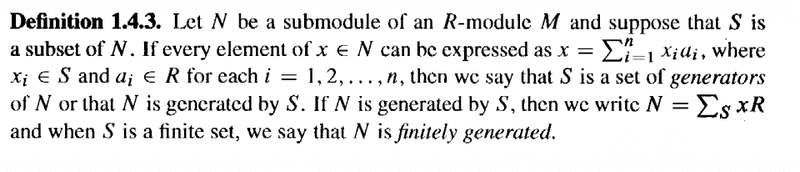#### Attachments

fresh_42
Mentor
2021 Award
Hi Andrew ... can you critique my proof of ##(1) \rightarrow (3)## please ... ... if I'm correct then the roof is trivial ... but ...

To show ##(1) \rightarrow (3)## ... ... using Bland's notation ...

Assume ##\{ x_\alpha \}## is a basis for ##M## ... ...

Then, for ##x \in M## we can write:

##x = \sum_\Delta x_\alpha a_\alpha## ... ... ... ... (1)

where ##\alpha \in \Delta## and only a finite number of ##a_i \neq 0## ... ...

But then we have that ##M = \bigoplus_\Delta x_\alpha R##

... ... because ... ...

##M = \bigoplus_\Delta x_\alpha R## means that any ##x \in M## can be expressed as ##x = \sum_\Delta x_\alpha a_\alpha##

(see notation regarding generators given below)

Is that OK?

Peter

*** NOTE ***

The following definition spells out notation that is relevant ... ...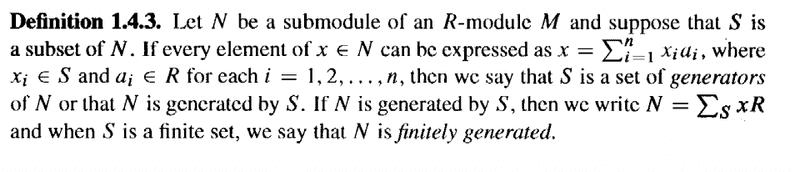This shows that you have a representation as a sum as required. But it leaves you with the question about the intersections. Those have to be zero for the sum to be called direct.
Why is ##x_\alpha R \cap x_\beta R = \{0\}##?

•Math Amateur
Gold Member
Thanks fresh_42 ... apreciate your help ...

Yes, need to attend to the issue of the intersections ...

Will do it shortly ...

Thanks again for the help ...

Peter

andrewkirk
Homework Helper
Gold Member
Hi MA. I'm travelling at present and only intermittently internet-connected. As Fresh said, your proof of the generation part is fine. For the intersection part, why not start with an element $$m\in bR\cap \bigoplus_{\substack{b'\in B\\b'\neq b}} b'R$$ and then use the linear independence feature of (1) to show that ##m## must be zero, which will give you the required intersection property to complete the conclusion of (3)?

Gold Member
Thanks Andrew ... yes, travelling myself ...

Left Southern Tasmania for regional Victoria ...

Will work on your suggestion when I get a moment ...

Peter

Gold Member
Thanks fresh_42 and Andrew ... for all your help ... it is much appreciated ...

... sorry to be slow in replying ... but travelling ... but now have Internet access in regional Victoria ...

As you both indicated, to prove that ##M = \bigoplus_\Delta x_{\alpha} R##

... we must establish the intersection condition ...

... that is ##x_\alpha R \ \cap \bigoplus_{ \beta \in \Delta \ \beta \neq \alpha } x_\beta R = 0##

So ... let ##m \in x_\alpha R \ \cap \bigoplus_{ \beta \in \Delta \ \beta \neq \alpha } x_\beta R ##

Then we have that ##m \in x_\alpha R## and ##m \in \bigoplus_{ \beta \in \Delta \ \beta \neq \alpha } x_\beta R ##

Now, since ##m \in \bigoplus_{ \beta \in \Delta \ \beta \neq \alpha } x_\beta R ## we have:

##m = x_{\beta_1} a_1 + x_{\beta_2} a_2 + x_{\beta_3} a_3 + ## ... ... ...

where ##\beta_i \in \Delta## and ##\beta_i \neq \alpha, a_i \in R ##

But in this sum each ##a_i## is unique ... so this means that ##m## cannot be expressed as ##m = x_\alpha a_\alpha## unless ##m = x_\alpha a_\alpha = 0## ... in other words m cannot belong, as is required, to ## x_\alpha R## unless m = 0 ... ...

Hence ##x_\alpha R \cap \bigoplus_{ \beta \in \Delta \ \beta \neq \alpha } x_\beta R = 0 ##

and so the required intersection condition is established ... ...

fresh_42, Andrew ... is the above proof OK ... I think it must be since I basically followed Andrew's advice ...

Thanks again to fresh_42 and Andrew ... ...

Peter

Last edited:
andrewkirk
Homework Helper
Gold Member
Gday MA.
In your proof you are using the uniqueness property, which is condition (2) rather than condition (1). Since the equivalence of 1 and 2 has already been proved, that is valid, but given that the initial aim was to prove that (1) entails (3), it seems to me that it would be more aesthetically pleasing to prove that without having to go via (2).

You should be able to use the fact that ##m## is in both ##x_\alpha R## and ##\bigoplus_{\substack{\beta\in\Delta\\\beta\neq\alpha}}x_\beta R## to write the difference of the two representations of ##m## in those two submodules as a finite linear combination of elements of ##\Delta## that is equal to zero. Then you can use the linear independence part of (1) to argue that all terms in the sum are zero, hence both representations (each of which is equal to ##m##) are zero.

By the way, did you know that, to get multiple lines in a subscript, like ##\bigoplus_{\substack{\beta\in\Delta\\\beta\neq \alpha}}## you can use the \substack command, writing

\bigoplus_{\substack{\beta \in \Delta \\ \beta \neq \alpha} }

The \\ escape code is a new-line command.
I find this very useful.
It looks even better in display format:

$$\bigoplus_{\substack{\beta \in \Delta \\ \beta \neq \alpha} }$$

Gold Member
Gday MA.
In your proof you are using the uniqueness property, which is condition (2) rather than condition (1). Since the equivalence of 1 and 2 has already been proved, that is valid, but given that the initial aim was to prove that (1) entails (3), it seems to me that it would be more aesthetically pleasing to prove that without having to go via (2).

You should be able to use the fact that ##m## is in both ##x_\alpha R## and ##\bigoplus_{\substack{\beta\in\Delta\\\beta\neq\alpha}}x_\beta R## to write the difference of the two representations of ##m## in those two submodules as a finite linear combination of elements of ##\Delta## that is equal to zero. Then you can use the linear independence part of (1) to argue that all terms in the sum are zero, hence both representations (each of which is equal to ##m##) are zero.

By the way, did you know that, to get multiple lines in a subscript, like ##\bigoplus_{\substack{\beta\in\Delta\\\beta\neq \alpha}}## you can use the \substack command, writing

\bigoplus_{\substack{\beta \in \Delta \\ \beta \neq \alpha} }

The \\ escape code is a new-line command.
I find this very useful.
It looks even better in display format:

$$\bigoplus_{\substack{\beta \in \Delta \\ \beta \neq \alpha} }$$

Thanks again for the help, Andrew ...

You write:

"... ... In your proof you are using the uniqueness property, which is condition (2) rather than condition (1). Since the equivalence of 1 and 2 has already been proved, that is valid, but given that the initial aim was to prove that (1) entails (3), it seems to me that it would be more aesthetically pleasing to prove that without having to go via (2) ... ... "

I agree ...

... so, as you indicate, a better proof would proceed as follows... ...

Require to show ##x_\alpha R \ \cap \bigoplus_{\substack{\beta \in \Delta \\ \beta \neq \alpha} } x_\beta R = 0##

So ... let ##m \in x_\alpha R \ \cap \bigoplus_{\substack{\beta \in \Delta \\ \beta \neq \alpha} } x_\beta R##

Then ... ... ##m \in x_\alpha R## and ##m \in \bigoplus_{\substack{\beta \in \Delta \\ \beta \neq \alpha} } x_\beta R ##

##\Longrightarrow \ m = x_\alpha a_\alpha## and ##m = \sum_{\substack{\beta \in \Delta \\ \beta \neq \alpha} } x_\beta R ##

##\Longrightarrow \ x_\alpha a_\alpha = \sum_{\substack{\beta \in \Delta \\ \beta \neq \alpha} } x_\beta R ##

##\Longrightarrow \ x_\alpha a_\alpha - \sum_{\substack{\beta \in \Delta \\ \beta \neq \alpha} } x_\beta R =0##

##\Longrightarrow \ a_i = 0## for all ##a_i \in \Delta## since ##\{ x_\alpha \}_\Delta## is a basis and consequently the ##x_\alpha## are linearly independent ... ...

## \Longrightarrow \ m = 0## and the intersection condition is proven ...

Is the proof satisfactory and correct?

Hope so ...

Peter

Last edited:
andrewkirk
Homework Helper
Gold Member
Yes that looks good.
Andrew

Gold Member
Gday MA.
In your proof you are using the uniqueness property, which is condition (2) rather than condition (1). Since the equivalence of 1 and 2 has already been proved, that is valid, but given that the initial aim was to prove that (1) entails (3), it seems to me that it would be more aesthetically pleasing to prove that without having to go via (2).

You should be able to use the fact that ##m## is in both ##x_\alpha R## and ##\bigoplus_{\substack{\beta\in\Delta\\\beta\neq\alpha}}x_\beta R## to write the difference of the two representations of ##m## in those two submodules as a finite linear combination of elements of ##\Delta## that is equal to zero. Then you can use the linear independence part of (1) to argue that all terms in the sum are zero, hence both representations (each of which is equal to ##m##) are zero.

By the way, did you know that, to get multiple lines in a subscript, like ##\bigoplus_{\substack{\beta\in\Delta\\\beta\neq \alpha}}## you can use the \substack command, writing

\bigoplus_{\substack{\beta \in \Delta \\ \beta \neq \alpha} }

The \\ escape code is a new-line command.
I find this very useful.
It looks even better in display format:

$$\bigoplus_{\substack{\beta \in \Delta \\ \beta \neq \alpha} }$$

I notice in my use of \bigoplus_{\substack{\beta \in \Delta \\ \beta \neq \alpha} } and \sum_{\substack{\beta \in \Delta \\ \beta \neq \alpha} }

that the ##\beta \in \Delta## and the ##\beta \neq \alpha## appeared to the side of the ##\bigoplus## and ##\sum## symbols ... ... how do you get them to appear underneath the main symbol ...

Peter

andrewkirk
The latex default is for subscripts to sums and similar operators to appear below and to the left of the operator for in-line latex and directly below the operator for display latex. Display latex is when the formula is on a line of its own, and is started and ended by . In-line latex is when the formula is on a line also containing text, which is started and ended by ## on physicsforums and by \$ or $$......$$ in standard latex.
•